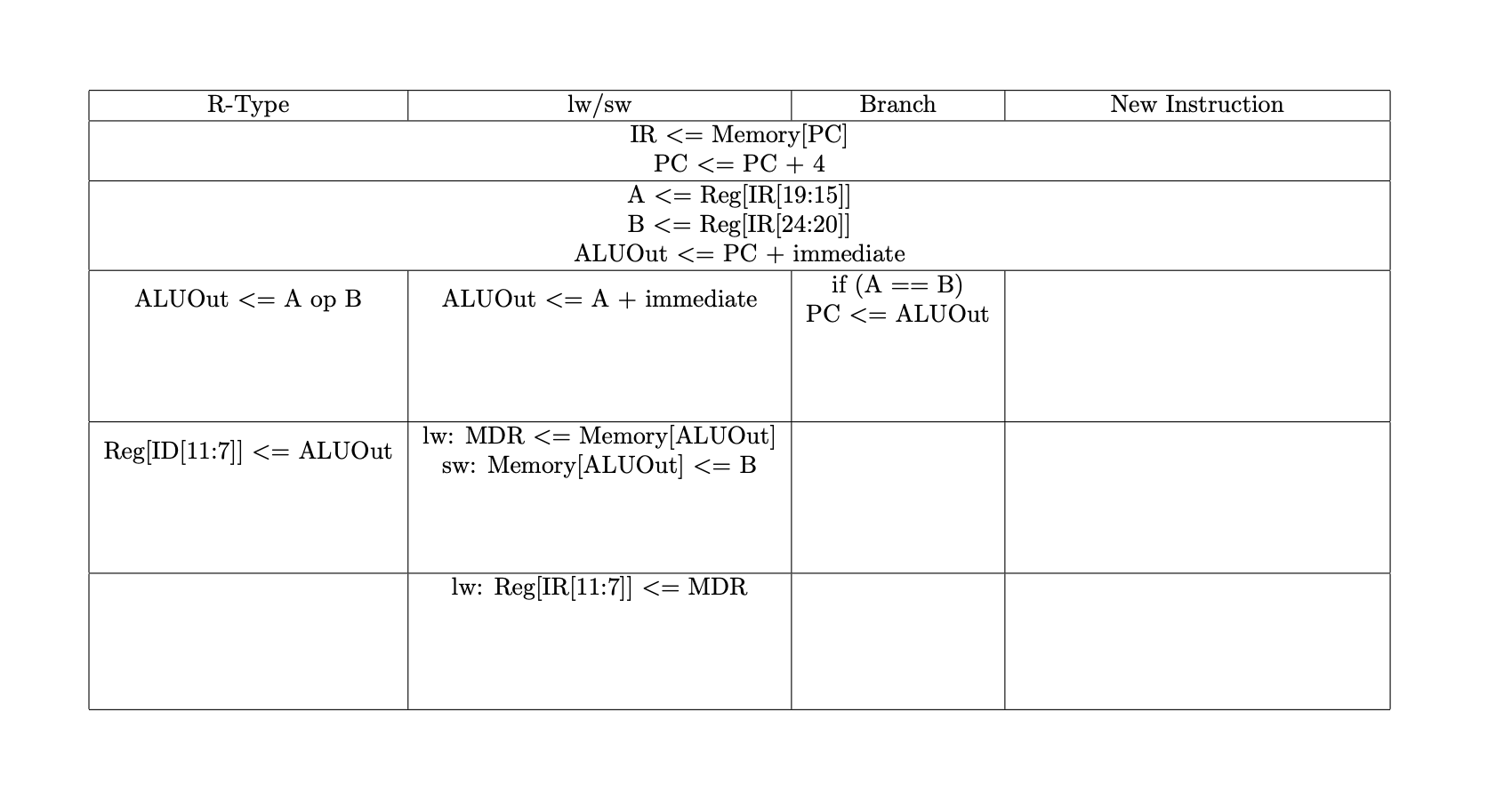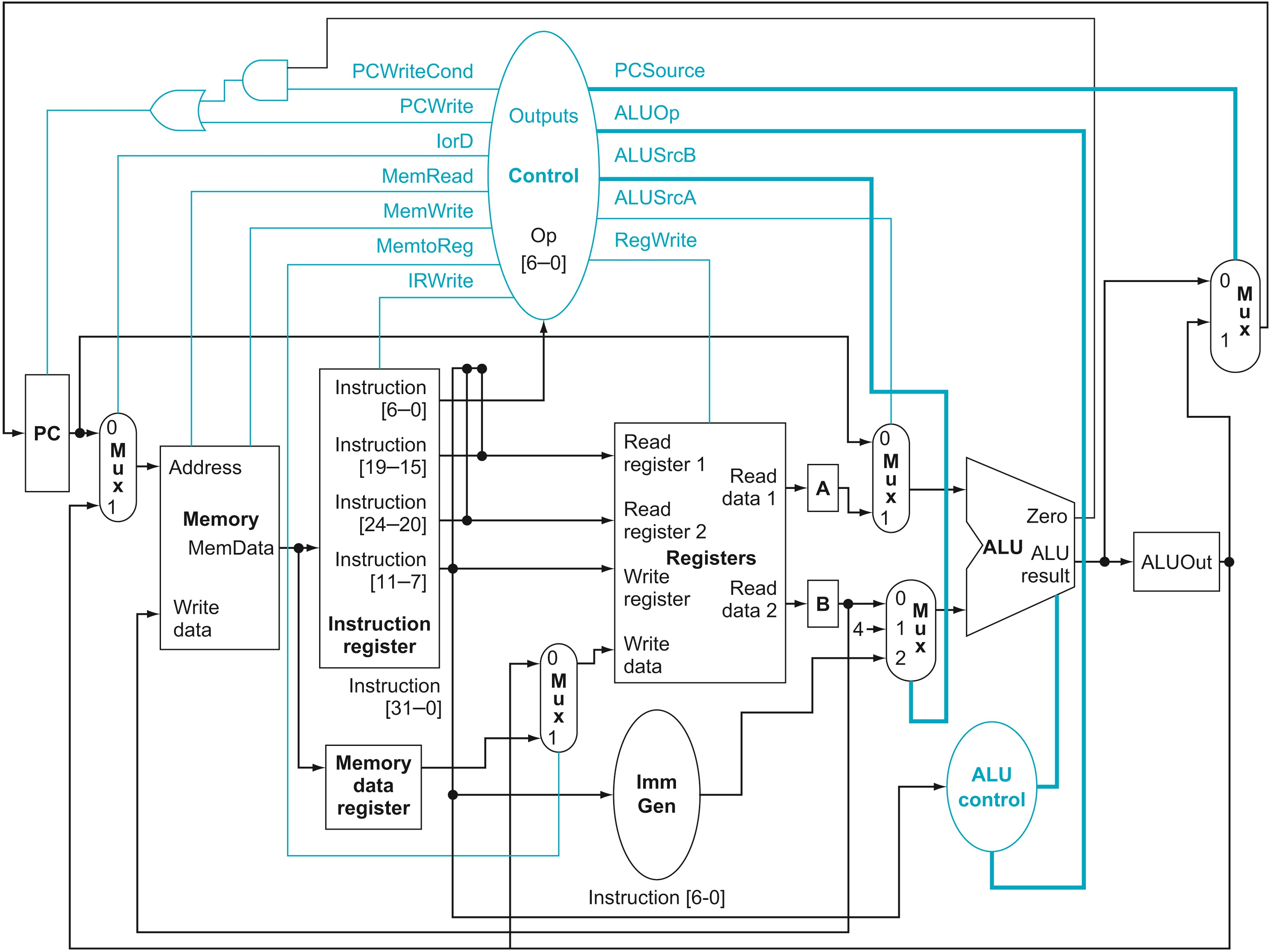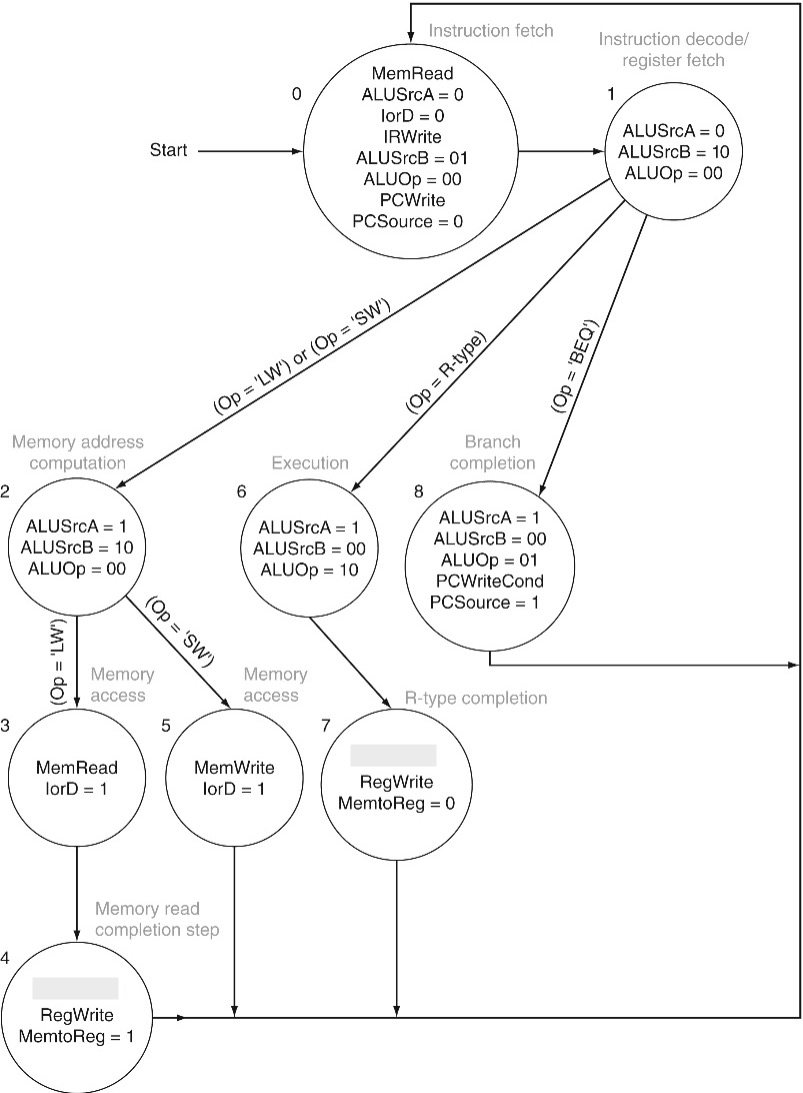Name: Box: Date:

## HW14 solution

1. (30 points) We wish to add a variant of the lw (load word) instruction, which increments the index register after loading the word from memory. This instruction (l_inc) corresponds to the following two instructions:

lw   $rt, L($rs)
addi $rs,$rs, 4

Modify the multi-cycle datapath to support the new instruction. Write the RTL for the new instruction below. Then, use the modify the attached multi-cycle datapath and control unit state diagrams. Your changes should be as minimal as possible.

The fastest and still minimal RTL is:

• Normal fetch
• Normal decode
• ALUout = A + SE(IR[15-0])
• MDR = Mem[ALUout]
• Reg[IR[20-16]] = MDR
ALUout = A + 4 #could be in previous cycle
• Reg[IR[25-21]] = ALUout

The datapath needs a new signal from IR[25-21] going into the RegDst mux. The control unit needs all RegDst signals updated to 2 bits, with a 0 MSB for all old signals and 10 allowing IR[25-21] to be the destination.

The control states are then:

• Standard fetch
• Standard decode
• If op=l_inc (could be combined with lw):
ALUsrcA = 1
ALUsrcB = 10
ALUop = 00
• MemRead
IorD = 1
• RegDst = 0
RegWrite
MemtoReg = 1
ALUsrcA = 1
ALUsrcB = 01
ALUop = 00
• RegDst = 10
RegWrite
MemtoReg = 0